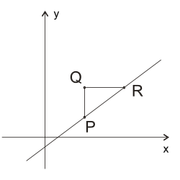## In the figure above, segments PQ and QR are each parallel to

##### This topic has expert replies
Moderator
Posts: 5631
Joined: 07 Sep 2017
Followed by:17 members

### In the figure above, segments PQ and QR are each parallel to

by BTGmoderatorDC » Sun Jan 20, 2019 12:11 am

00:00

A

B

C

D

E

## Global StatsIn the figure above, segments PQ and QR are each parallel to one of the coordinate axes. What is the ratio of the length of QR to PQ?

(1) The slope of the line that passes through points P and R is 0.75.
(2) The coordinates of point P are (4,2).

OA A

Source: Ecpnomist GMAT

### GMAT/MBA Expert

GMAT Instructor
Posts: 3008
Joined: 22 Aug 2016
Location: Grand Central / New York
Thanked: 470 times
Followed by:32 members
by [email protected] » Sun Jan 20, 2019 4:03 am

00:00

A

B

C

D

E

## Global Stats

BTGmoderatorDC wrote:In the figure above, segments PQ and QR are each parallel to one of the coordinate axes. What is the ratio of the length of QR to PQ?

(1) The slope of the line that passes through points P and R is 0.75.
(2) The coordinates of point P are (4,2).

OA A

Source: Ecpnomist GMAT
We have to get the value of the ratio of the length of QR to PQ.

Let's take each statement one by one.

(1) The slope of the line that passes through points P and R is 0.75.

Say the coordinates of points P are (x1, y1) and the coordinates of points R are (x2, y2); thus, the coordinates of points Q would be (x1, y2).

Thus, QR = (x2 - x1) and QP = (y2 - y1)

Thus, QR/QP = (x2 - x1)/(y2 - y1)

It is given that the slope of the line that passes through points P and R is 0.75; thus, QP/QR = slope = (y2 - y1)/(x2 - x1) = 0.75

Thus, QR/QP = 1/0.75 = 4/3. Sufficient.

(2) The coordinates of point P are (4,2).

We do not have information about x2 and y2. Insufficient.

The correct answer: A

Hope this helps!

-Jay
_________________
Manhattan Review

Locations: Manhattan Review Kukatpally | GMAT Prep Jayanagar | GRE Prep Tarnaka | Madhapur GRE Coaching | and many more...

Schedule your free consultation with an experienced GMAT Prep Advisor! Click here.

### GMAT/MBA Expert

GMAT Instructor
Posts: 2094
Joined: 04 Dec 2012
Thanked: 1443 times
Followed by:245 members
by ceilidh.erickson » Mon Jan 21, 2019 1:59 pm

00:00

A

B

C

D

E

## Global Stats

It is generally true in Data Sufficiency that if the question asks you for a PROPORTION, you do not need concrete values to answer it.

In this case, we're asked for a RATIO of horizontal and vertical lengths, but not for actual values. We can think of any slope as a RATIO of vertical to horizontal (rise/run). For example, a slope of 2/3 means "rise 2, run 3." We could go from (0, 0) to (2, 3) to (4, 6) to (8, 9), etc. Between any two of these points, the ratio of the vertical distance to the horizontal distance will be 2 to 3.

Thus, when the question asks "What is the ratio of the length of QR to PQ?", we could rephrase as:
what is the slope of line PR?

-----------------------------------------------------------------------------------------
(1) The slope of the line that passes through points P and R is 0.75.

This answers our target question. Sufficient.
-----------------------------------------------------------------------------------------
(2) The coordinates of point P are (4,2).

A single set of values tells us nothing about proportions. Insufficient.
-----------------------------------------------------------------------------------------
The answer is A.
Ceilidh Erickson
EdM in Mind, Brain, and Education
Harvard Graduate School of Education

• Page 1 of 1